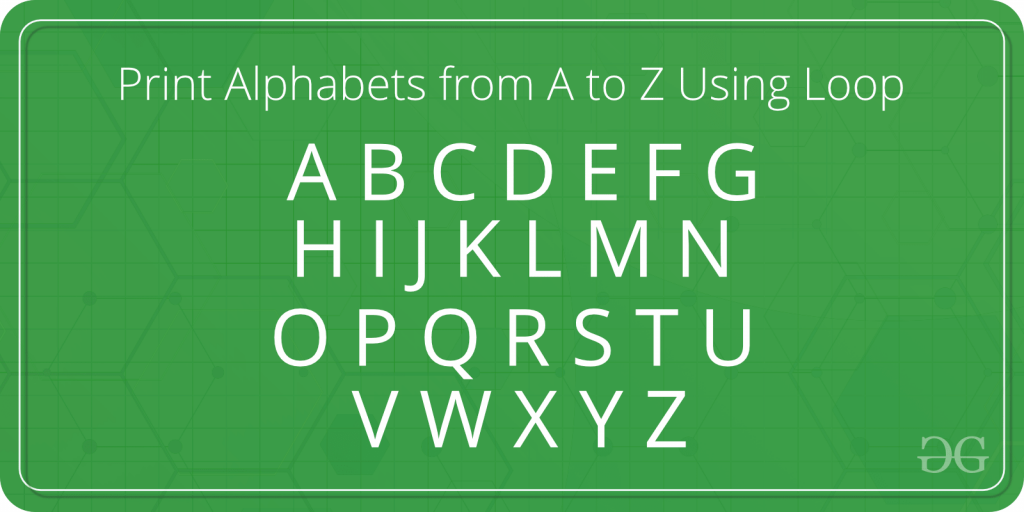# Program to Print Alphabets from A to Z Using LoopThe task is to print the alphabets from A to Z using a loop.

## Recommended: Please try your approach on {IDE} first, before moving on to the solution.

In the below program, for loop is used to print the alphabets from A to Z. A loop variable is taken to do this of type ‘char’. The loop variable ‘i’ is initialized with the first alphabet ‘A’ and incremented by 1 on every iteration. In the loop, this character ‘i’ is printed as the alphabet.

Program:

## C++

 `// C++ program to find the print ` `// Alphabets from A to Z ` `#include ` `using` `namespace` `std; ` ` `  `int` `main() ` `{ ` `    ``// Declare the variables ` `    ``char` `i; ` ` `  `    ``// Display the alphabets ` `    ``cout << ``"The Alphabets from A to Z are: \n"``; ` ` `  `    ``// Traverse each character ` `    ``// with the help of for loop ` `    ``for` `(i = ``'A'``; i <= ``'Z'``; i++) ` `    ``{ ` ` `  `        ``// Print the alphabet ` `        ``cout << i <<``" "``; ` `    ``} ` ` `  `    ``return` `0; ` `} ` ` `  `// This code is contributed by ` `// Shubhamsingh10 `

## C

 `// C program to find the print ` `// Alphabets from A to Z ` ` `  `#include ` ` `  `int` `main() ` `{ ` `    ``// Declare the variables ` `    ``char` `i; ` ` `  `    ``// Display the alphabets ` `    ``printf``(``"The Alphabets from A to Z are: \n"``); ` ` `  `    ``// Traverse each character ` `    ``// with the help of for loop ` `    ``for` `(i = ``'A'``; i <= ``'Z'``; i++) { ` ` `  `        ``// Print the alphabet ` `        ``printf``(``"%c "``, i); ` `    ``} ` ` `  `    ``return` `0; ` `} `

## Java

 `// Java program to find the print ` `// Alphabets from A to Z ` `class` `GFG ` `{ ` ` `  `    ``public` `static` `void` `main(String[] args)  ` `    ``{ ` `        ``// Declare the variables ` `        ``char` `i; ` ` `  `        ``// Display the alphabets ` `        ``System.out.printf(``"The Alphabets from A to Z are: \n"``); ` ` `  `        ``// Traverse each character ` `        ``// with the help of for loop ` `        ``for` `(i = ``'A'``; i <= ``'Z'``; i++) ` `        ``{ ` ` `  `            ``// Print the alphabet ` `            ``System.out.printf(``"%c "``, i); ` `        ``} ` ` `  `    ``} ` `} ` ` `  `/* This code contributed by PrinciRaj1992 */`

## Python3

 `# Python3 program to find the print ` `# Alphabets from A to Z ` ` `  `if` `__name__ ``=``=` `'__main__'``: ` `     `  `    ``# Declare the variables ` `    ``i ``=` `chr``; ` ` `  `    ``# Display the alphabets ` `    ``print``(``"The Alphabets from A to Z are: "``); ` ` `  `    ``# Traverse each character ` `    ``# with the help of for loop ` `    ``for` `i ``in` `range``(``ord``(``'A'``), ``ord``(``'Z'``) ``+` `1``): ` ` `  `        ``# Print the alphabet ` `        ``print``(``chr``(i), end``=``" "``); ` `         `  `# This code is contributed by Rajput-Ji `

## C#

 `// C# program to find the print ` `// Alphabets from A to Z ` `using` `System; ` ` `  `class` `GFG ` `{ ` ` `  `    ``public` `static` `void` `Main(String[] args)  ` `    ``{ ` `        ``// Declare the variables ` `        ``char` `i; ` ` `  `        ``// Display the alphabets ` `        ``Console.Write(``"The Alphabets from A to Z are: \n"``); ` ` `  `        ``// Traverse each character ` `        ``// with the help of for loop ` `        ``for` `(i = ``'A'``; i <= ``'Z'``; i++) ` `        ``{ ` ` `  `            ``// Print the alphabet ` `            ``Console.Write(``"{0} "``, i); ` `        ``} ` ` `  `    ``} ` `} ` ` `  `// This code is contributed by Rajput-Ji `

Output:

```The Alphabets from A to Z are:
A B C D E F G H I J K L M N O P Q R S T U V W X Y Z
```

My Personal Notes arrow_drop_upCheck out this Author's contributed articles.

If you like GeeksforGeeks and would like to contribute, you can also write an article using contribute.geeksforgeeks.org or mail your article to contribute@geeksforgeeks.org. See your article appearing on the GeeksforGeeks main page and help other Geeks.

Please Improve this article if you find anything incorrect by clicking on the "Improve Article" button below.

Article Tags :

Be the First to upvote.

Please write to us at contribute@geeksforgeeks.org to report any issue with the above content.